# HSSlive: Plus One & Plus Two Notes & Solutions for Kerala State Board

## AP Board Class 7 Maths Chapter 6 Data Handling InText Questions Textbook Solutions PDF: Download Andhra Pradesh Board STD 7th Maths Chapter 6 Data Handling InText Questions Book AnswersAP Board Class 7 Maths Chapter 6 Data Handling InText Questions Textbook Solutions PDF: Download Andhra Pradesh Board STD 7th Maths Chapter 6 Data Handling InText Questions Book Answers

## Andhra Pradesh State Board Class 7th Maths Chapter 6 Data Handling InText Questions Books Solutions

 Board AP Board Materials Textbook Solutions/Guide Format DOC/PDF Class 7th Subject Maths Chapters Maths Chapter 6 Data Handling InText Questions Provider Hsslive

2. Click on the Andhra Pradesh Board Class 7th Maths Chapter 6 Data Handling InText Questions Answers.
3. Look for your Andhra Pradesh Board STD 7th Maths Chapter 6 Data Handling InText Questions Textbooks PDF.
4. Now download or read the Andhra Pradesh Board Class 7th Maths Chapter 6 Data Handling InText Questions Textbook Solutions for PDF Free.

## AP Board Class 7th Maths Chapter 6 Data Handling InText Questions Textbooks Solutions with Answer PDF Download

Find below the list of all AP Board Class 7th Maths Chapter 6 Data Handling InText Questions Textbook Solutions for PDF’s for you to download and prepare for the upcoming exams:

Where does the Arithmetic Mean lie ?
The marks obtained by Sarala, Bindu, Geeta and Rekha in Telugu, Hindi and English are given below.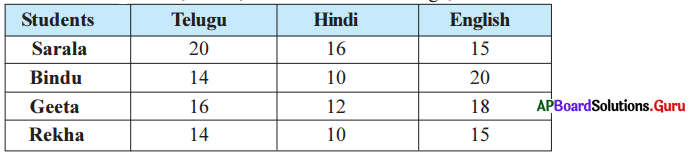Now, let us calculate the average marks obtained by the students in each subject.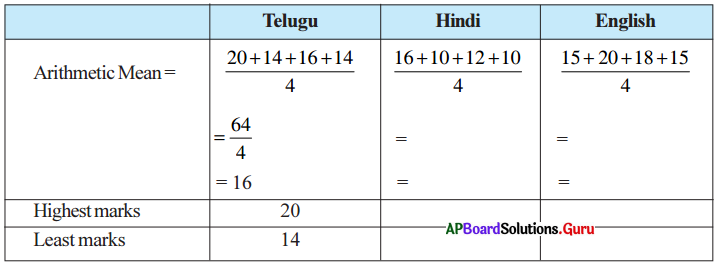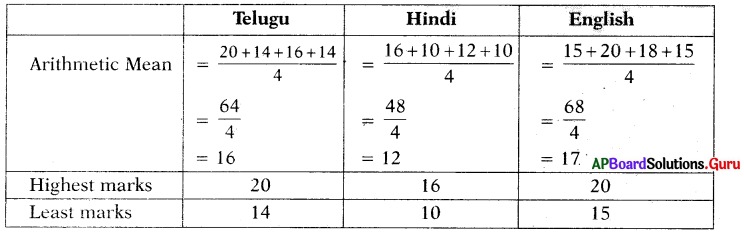(i) What do you observe from the above table ?
Mean always lie between the maximum and minimum values/observations of the data.

(ii) Does the mean lies between maximum and minimum values in each case ?
Yes, Its always true.
i.e., Mean always lies between the maximum and minimum values of the data.

Check Your Progress [Page No: 109]

Question 1.
Find the Arithmetic Mean of first three multiples of 5 ?
Given, first three multiples of 5 are: 5, 10, 15.
Sum of observations = 5 + 10 + 15 = 30
Number of observations = 3
Arithmetic mean = Sum of observations  Number of observations  = 303 = 10
∴ Arithmetic mean of first three multiples of 5 is 10.

Let’s Explore [Page No. 109]

Collect the information about the weights of any ten students of your class in kilograms arid answer the following: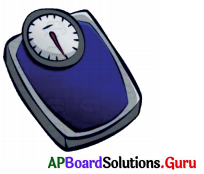Name of the student Weight in kilograms Aditya 38 Kishore 39 Balu 40 Sreeja 36 Sreekari 35 Kairavi 3.7 Swathi 36 Kirshna 41 Ram 39 Prasad 39

Question 1.
What are the greatest and smallest weights ?
Krishna = 41 kg – Greatest weight
Sreekari = 35 kg – Smallest weight

Question 2.
Find Arithmetic mean of collected data.
Given observations: 38, 39, 40, 36, 35, 37, 36, 41, 39, 39.
Sum of observations = 38 + 39 + 40 + 36 + 35 + 37 + 36 + 41 + 39 + 39 = 380
Number of observations = 10
Arithmetic Mean =  Sum of observations  Number of observations  = 38010 = 38 kg.
Number of observations 10
∴ Arithmetic mean weight of 10 students of our class is 38 kg.

Question 3.
Verify whether Arithmetic Mean lies between greatest and smallest observations or not.
Yes, Arithmetic mean 38 kg lies between greatest weight 41 kg and smallest weight 35 kg.

Check Your Progress [Page No. 110]

Question 1.
What is the range of first ten whole numbers?
First ten wh4’e numbers are 0, 1, 2, 3, 4, 5, 6, 7, 8, 9.
Maximum value = 9
Minimum value = 0
Range = Maximum Value – Minimum Value = 9 – 0 = 9
∴ Range = 9

Check Your Progress [Page No. 111]

Find the mode of 10, 9, 12, 10, 8, 7, 6, 10, 9, 7, 8, 5 and 2.
Given data : 10, 9, 12, 10, 8, 7, 6, 10, 9, 7, 8, 5 and 2.
By arranging the numbers with same values together.
2, 5, 6, 7, 7, 8, 8, 9, 9, 10, 10, 10, 12
As ’10’ occurs more frequently than other observations.
∴ Mode = 10

Let’s Explore [Page No. 111]

Take a dice, roll it 20 times and record the numbers you got on its top face. Find the ‘Mode’ of resulting numbers.
A dice rolled 20 times and the numbers are
2, 4, 5, 3, 1, 6, 5, 4, 2, 1, 3, 5, 4, 2, 6, 2, 2, 5, 1, 3.
By arranging the numbers with same values together
1, 1, 1, 2, 2, 2, 2, 2, 3, 3, 3, 4, 4, 4, 5, 5, 5, 5, 6, 6.
As 2 occurs more frequently than other observations.
Mode = 2.

Let’s Think [Page No: 111]

The following data shows that the number of hours spent by students for study. Find the mode: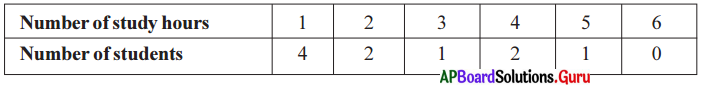In the given data more number of students spend 1 hour for study.
So, mode of the data = 4.

Check Your Progress [Page No: 114]

What is the median of first 7 prime numbers ?
First 7 prime numbers are 2, 3, 5, 7, 11,13, 17.
Arrange the given observations in ascending order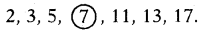In seven observations the 4th observation 7 is the middle most value.
∴ Median = 7.

Let’s Explore [Page Mo. 114]

Question 1.
Collect 10th class pass percentage for last six years of your school (or) your near by school. Find median of the data.
10th Class pass percentage for last six years of our school are: 100%, 98%, 93%, 95%, 96%, 97%.
Arrange the given observations in ascending order.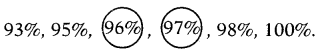In six observations the 3rd and 4th observations are 96% and 97%.
Here, we have two middle most values 96% and 97%.
Median = Average of two middle most values
96+972 = 96.5%
∴ Median of the data = 96.5%

Project Work [Page No: 114]

Visit any vegetable market along with your parent. Collect the information about the costs of different vegetables. By using this data fill the following table.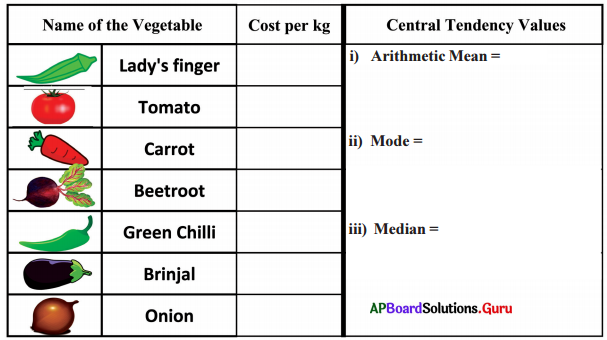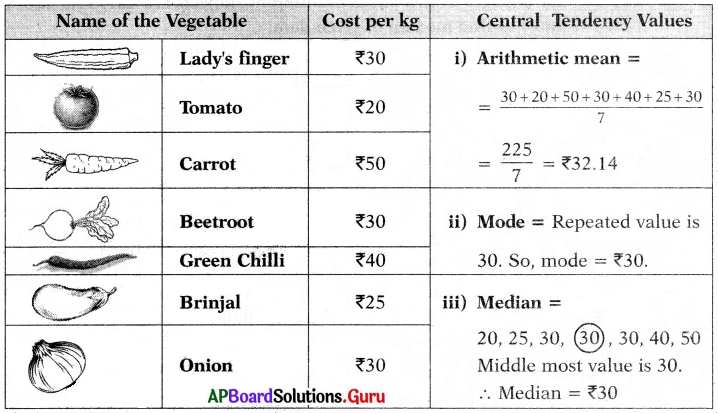[Page No. 115]

Prasanna wanted to buy a mobile phone. He selected two mobile phones of different companies having same features. Now he would like to know which mobile phone has good performance. He collected the information of star rating from different magazines and news papers.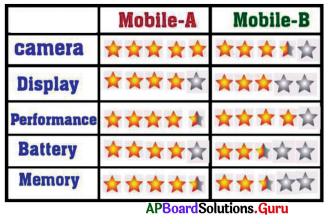Question 1.
What information is given in the table ?
The table is showing information about the mobile phones.

Question 2.
Is the above information being useful to Prasanna ?
Yes.

Question 3.
Which mobile phone you will suggest to Prasanna ?
Mobile A. (21 + 12 + 12 rating)

Check Your Progress [Page No. 116]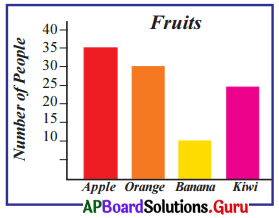Question 1.
Which fruit most of the people like ?
Most of the people liked Apple.

Question 2.
How many people likes banana?
10.

Observe the following graph. [Page No. 116]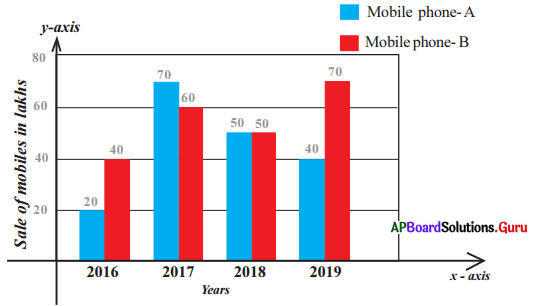Observe the above double bar graph and answer the following:

Question 1.
In which year the sales of both mobile phone companies are equal ?
2018.

Question 2.
In 2017, which mobile phone company has more sales ?
Mobile phone – A.

Examples

Question 1.
Mid Day Meal (MDM) taken by the students in six days are 132, 164, 145, 182, 163 and 114, Find Arithmetic Mean of students who took MDM per day.
Given, number of students who took MDM 132, 164, 145, 182, 163, 114.
Arithmetic Mean =  Sum of observations  Number of observations
132+164+145+182+163+1146
9006
= 150

Question 2.
Ages of students (in years) are 8, 5, 6, 6, 5, 7, 5, 6, 5, 4 7, 6, 7, 6, 5 8 and 6 find the mode of given data.
Given, ages of students are 8, 5, 6, 6, 5, 7, 5, 6, 5, 4, 7, 6, 7, 6, 5, 8, 6.
By arranging the numbers with same values together
4, 5, 5, 5, 5,5,6, 6,6,6, 6, 6,7,7, 7, 8, 8.
As 6’ occurs more frequently than other observations.
∴ Mode = 6

Question 3.
Find the mode of the data of Grades A, B, E, A, C, E, B, C, D, A, D, C, F, A and C.
Given, A, B, E, A, C, E, B, C, D, A, D, C, F, A, C.
By arranging the letters of same type together
A, A, A, A, B, B, C, C, C, C, D, D, E, E, F
As ‘A’ and ‘C’ occurs most frequently in the data.
∴ Mode = A and C

Question 4.
Find the median of data 32, 43, 25, 67, 46, 71 and 182.
Given 32, 43, 25, 67, 46, 71, 182
Arrange the given observations in ascending order.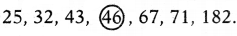In ‘7’ observations the 4th observation is the middle most value.
∴ Median = 46.

Question 5.
The monthly incomes of 8 members are ₹8000, ₹9000, f8200, ₹7900, ₹8500, ₹8600, ₹7700 and ₹60000. Find the median income.
Given that, the monthly incomes of 8 members are:
₹8000, ₹9000, ₹8200, ₹7900, ₹8500, ₹8600, ₹7700, ₹60000
Arrange the given observations in ascending order.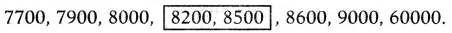Here, we have two middle most values 8200 and 8500.
In this case, Median = Average of two middle most values
8200 + 8500 16700
8200+85002 = 167002 = ₹8,350

Question 6.
The number of CFL bulbs and LED bulbs by a seller every month from March to August are given below. Draw by vertical double bar graph.

 Month CFL Bulbs LED Bulbs March 70 75 April 35 30 May 65 75 June 90 100 July 22 35 August 50 50

Steps in drawing a Double bar graph:

1. Draw X-axis (horizontal line) and Y – axis (vertical line) on the graph paper and mark their intersection point as ‘O’.
2. Take ‘months’ on X – axis.
3. Take number of CFL and LED bulbs on Y ~ axis.
4. Take an appropriate scale on Y-axis so that number of both the bulbs can be shown easily. Here the maximum value to be plotted on Y – axis as 100, so let us take 1 cm =10 bulbs on Y – axis.
5. Find the length of each bar by dividing the value by 10 (as scale is 1 cm = 10 bulbs).

Eg: Length of bar represents 70 CFL bulbs = 7010 = 7 cm
Length of bar represents 75 LED bulbs = 7510 = 7.5 cm

Question 6.
Draw the bars of uniform width representing ‘CFL bulb’ and ‘LED bulb’ side by side of every month.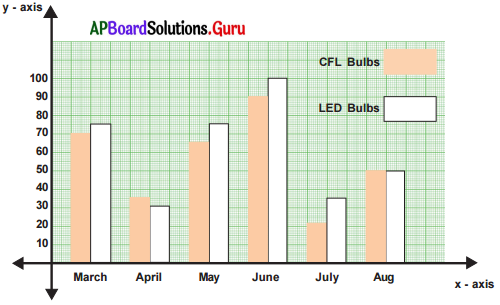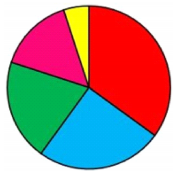Question 1.
Largest portion of the circle is shaded by which colour ?
Red.

Question 2.
Are the portions of circle shaded by blue and pink are in same size ?
No.

Question 3.
Smallest portion of the circle is shaded by which colour ?
Yellow.

Question 2.
Observe the below picture which shows various expenses of Manasa’s family: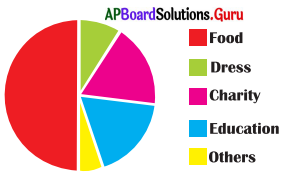(i) For which item highest amount spent?
Food.

(ii) For which items same amount spent?
Charity and Education.

(iii) For which item least amount spent?
Others.

Question 7.
In a school, there are 100 students in class VII and every student is a member of any one of the club. The following table shows the number of students in various clubs, then Construct the pie chart to following data:

 Club Number of members Mathematics 50 Science 30 Social Studies 40 English 40 Arts 20

The angle of each sector will depend on the ratio between the number of students in club and total number of students.
Angle of sector =  Value of the item  Sum of the values of all items  × 360°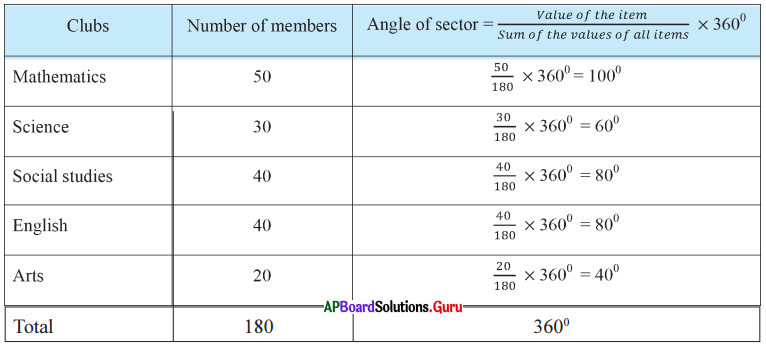Steps of construction:

1. Draw a circle with any convenient radius and mark it’s centre as ‘O’.
2. Mark a point A, somewhere on the circumference and join OA.
3. Construct ∠AOB = 100° to represent angle of the sector for Maths club.
4. Construct ∠BOC = 60° to represent angle of the sector for Science club.
5. Construct ∠COD = 80° to represent angle of the sector for Social Studies club.
6. Construct ∠DOE = 80° to represent angle of the sector for English club.
7. Now ∠EOA = 40° to represent angle of the sector for Arts club.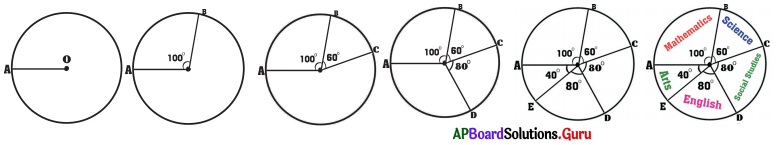Practice Questions [Page No: 124]

In the following alphabetical series, a term (next term) is missing. Choose the missing term from the options.

Question 1.
B, F, J, N, R,’V, …..
(a) Z
(b) W
(c) X
(d) Y
(a) Z

Explaination: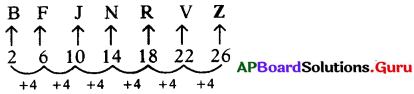Question 2.
A, C, E, G, I, K, …..
(a) P
(b) O
(c) N
(d) M
(d) M

Explaination: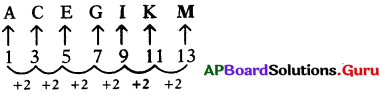Question 3.
M, O, R, T, …….
(a) W
(b) U
(c) V
(d) Q
(a) W

Explaination: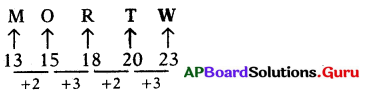Question 4.
U, S, P, L, …….
(a) F
(b) G
(c) H
(d) I
(b) G

Explaination: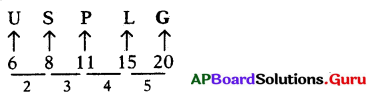Question 5.
ZA, YB, XC, WD,
(a) UE
(b) EV
(c) VE
(d) SH
(c) VE

Explaination: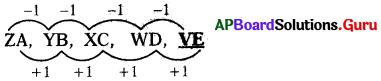Question 6.
AM, BO, CQ, DS, EU, …………
(a) WF
(b) FU
(c) GV
(d) FW
(d) FW

Explaination: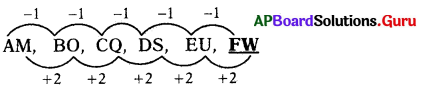In the series next word is FW.

Question 7.
ZY, XV, UR, QM, …..
(a) LG
(b) LI
(c) LH
(d) KJ
(a) LG

Explaination: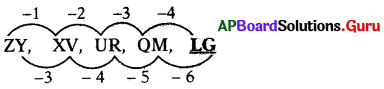So, in the series next word is LG.

Question 8.
AC, DF, GI, JL, …..
(a) NO
(b) MO
(c) MN
(d) NP
(b) MO

Explaination: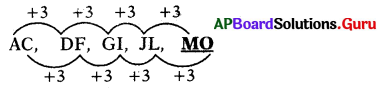So, in the series next word is MO.

Question 9.
DN, EM, FL, GK, HJ, …..
(a) IK
(b) GI
(c) IJ
(d) II
(d) II

Explaination: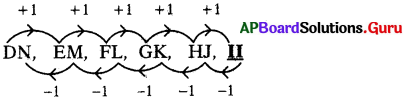So, in the series next word is II.

Question 10.
CBA, STU, FED, VWX,
(a) IHG
(b) GHI
(c) IJK
(d) YZA
(a) IHG

Explaination: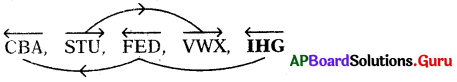So, in the series next word is IHG.

Question 11.
AZC, DYF, GXI, JWL
(a) OVM
(b) UNV
(c) MVO
(d) MNO
(c) MVO

Explaination: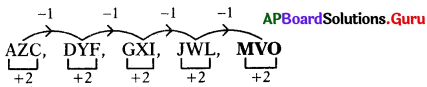So, in the series next word is MVO.

Question 12.
ABK, CDL, EFM, GHN,
(a) JIO
(b) IJO
(c) MNO
(d) ONM
(b) IJO

Explaination: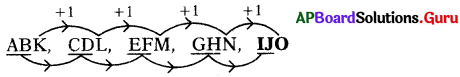So, in the series next word is IJO.

Question 13.
A2C, D5F, G8I, J11L, …..
(a) Ml40
(b) Ml20
(c) N15P
(d) N12P
(a) Ml40

Explaination: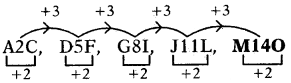So, in the series next word is M140.

Question 14.
A, CD, HIJ, PQRS, …………..
(a) ZABCD
(b) ZYXW
(c) ABCDE
(d) RSTUV
(c) ABCDE

Explaination: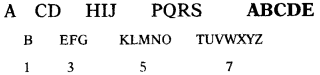So, in the series next word is ABCDE.

Question 15.
A, BC, DEF, GHIJ,
(a) KLMNP
(b) LMNOP
(c) KLMNO
(d) JKLMN
(c) KLMNO

Explaination:
A BC DEF GHIJ KLMNO
So, in the series next word is KLMNO.

## Andhra Pradesh Board Class 7th Maths Chapter 6 Data Handling InText Questions Textbooks for Exam Preparations

Andhra Pradesh Board Class 7th Maths Chapter 6 Data Handling InText Questions Textbook Solutions can be of great help in your Andhra Pradesh Board Class 7th Maths Chapter 6 Data Handling InText Questions exam preparation. The AP Board STD 7th Maths Chapter 6 Data Handling InText Questions Textbooks study material, used with the English medium textbooks, can help you complete the entire Class 7th Maths Chapter 6 Data Handling InText Questions Books State Board syllabus with maximum efficiency.

## FAQs Regarding Andhra Pradesh Board Class 7th Maths Chapter 6 Data Handling InText Questions Textbook Solutions

#### Can we get a Andhra Pradesh State Board Book PDF for all Classes?

Yes you can get Andhra Pradesh Board Text Book PDF for all classes using the links provided in the above article.

## Important Terms

Andhra Pradesh Board Class 7th Maths Chapter 6 Data Handling InText Questions, AP Board Class 7th Maths Chapter 6 Data Handling InText Questions Textbooks, Andhra Pradesh State Board Class 7th Maths Chapter 6 Data Handling InText Questions, Andhra Pradesh State Board Class 7th Maths Chapter 6 Data Handling InText Questions Textbook solutions, AP Board Class 7th Maths Chapter 6 Data Handling InText Questions Textbooks Solutions, Andhra Pradesh Board STD 7th Maths Chapter 6 Data Handling InText Questions, AP Board STD 7th Maths Chapter 6 Data Handling InText Questions Textbooks, Andhra Pradesh State Board STD 7th Maths Chapter 6 Data Handling InText Questions, Andhra Pradesh State Board STD 7th Maths Chapter 6 Data Handling InText Questions Textbook solutions, AP Board STD 7th Maths Chapter 6 Data Handling InText Questions Textbooks Solutions,
Share: